# Maths and Physics➝The Physics Cube

The universe is goverened by three fundamental constants: The speed of light, c, Planck's constant, h, and Newton's gravitational constant, G. Advances in theoretical physics can be seen as a journey around a cube representing which of these constants we choose to include in our theories and which we choose to ignore. The ultimate goal is to include all three constants consistently in a theory of quantum gravity.

If you are using a compatible browser you should see below a representation of this cube. You can click on the cube and drag to rotate it. As you bring to the front whichever physical theory catches your eye, a brief explanation of the theory will be displayed below.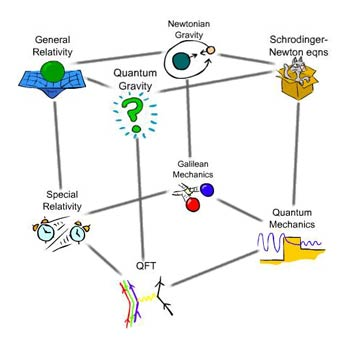If we are in a situation where the gravitational field is weak enough to ignore, things are moving slow compared to the speed of light and the mysterious world of the atom too tiny to be of importance, then we are living in a world governed by Galilean mechanics. Effectively, we have set Newton's constant and Planck's constant to zero, and at the same time sent the speed of light to infinity.

This is the physics we are all familiar with from school. Here objects are at a definite position and moving with a definite velocity. Here, when you walk at 5mph along a train moving at 70mph you'll be moving at 75mph. The most exciting thing here is the fact that this is not how the real world works.One of Newton's many great achievements was his universal law of gravitation. He stated that every body in the universe attracts every other body with a force proportional to the product of their masses and inversely proportional to the square of their separation. The constant of proportionality is known as Newton's gravitational constant, G, and in this corner of the cube it is the only constant that is considered.The idea that the speed of light, c, is a finite constant for all observers, no matter what their velocity may seem somewhat contradictory. For one, it is never possible to catch up with a beam of light if its velocity is always the same relative to you. How can the beam of light from a car headlights be receeding away from the car at the same speed as they receed away from a man standing still on the pavement? These apparent contradictions were resolved by Einstein with his Special Theory of Relativity. The solution was to realise that it is our assumptions about space and time that need to be altered.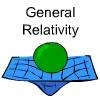In order to unify Newtonian gravity with the Special Theory of Relativity what was needed was a theory which succesfully encompassing both the constants G and c. This great achievement was realised by Einstein's General Theory of Relativity.

Einstein realised that the occupants of a freely falling elevator - before hitting the ground - may well consider themselves equally to be in the far depths of space away from the pull of any gravitational field. Further, the occupants of an elevator stuck between floors may well consider themselves in the far depths of space away from any gravitational pull, yet with a rocket strapped underneath the elevator, accelerating it uniformly.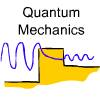The strange world of quantum mechanics is motivated by experiment, and experiment has shown us that when we begin to probe the realm of the atom, things get really weird. Here a new constant comes into play, Planck's constant, h.

The physics here is governed by the Schrödinger equation. Here, the state of a particle is described by a wave function. The amplitude of the wave at a given point determines the probability that, upon measurement, the particle will be found to be at that point. This probabilistic behaviour is fundamental to measurement in quantum mechanics and leads to many counterintuitive phenomena.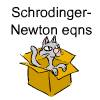This is perhaps the least studied corner of the cube. By trying to understand how a theory can encompass the two constants G and h, it is hoped some light will be shed on the full theory of quantum gravity. The theory involves solving the Schrödinger equation for a particle moving in a potential, however the potential is not some arbitrary fixed background as in ordinary quantum mechanics but is rather the gravitational potential of the particle itself (or rather its probability density). This leads to interesting and complicated nonlinear behaviour.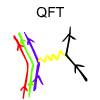The construction of a theory consitent with both special relativity and quantum mechanics was at first frought with problems of negative energies. However, it was realised that these problems could be reinterpreted, and with this came the prediction of antimatter. This was an early success for Quantum Field Theory, which has since developed to describe the atomic world to an astonishing accuracy through what is known as the Standard Model.The as yet undiscovered holy grail of modern physics. A theory which succesfully encompasses the three fundamental constants G, h and c. There are a couple of young pretenders to this throne, String Theory and Loop Quantum Gravity, however neither theory can yet definitevely say that it is correct, and in the end they may both be wrong.

By combining the three constants G, h and c in different ways we can construct fundamental units of length, time and energy, the so called Planck units. It is at scales comparable to these Planck scales that the theory of quantum gravity is expected to become important. The unfortunate thing is that these scales are currently far beyond the reach of our experiments, for instance the Planck length is approximately 10-35 metres, which is a massive 1020 times smaller than the proton.